Latest Banking jobs   »

# Quantitative Aptitude Quiz For Bank Foundation 2023 -02nd May

Q1. A and B entered into a partnership. They invested for same period of time and B got Rs 5250 as his share in profit. If ratio between total investment of A and B together to total profit is 28 : 15, then find B’s investment
(a) Rs. 9100
(b) Rs. 9800
(c) Rs. 8400
(d) Rs. 11200
(e) Rs. 12600

Q2. Anurag and Roshan started a business with capital Rs. 12,000 and Rs. 14000. After 6-month Roshan withdraws 25% of his investment. If the annual profit of business was Rs. 9,700 then find the profit share of Anurag.
(a) Rs. 4,000
(b) Rs. 3,200
(c) Rs. 4,500
(d) Rs. 4,800
(e) Rs. 5,400

Q3. A and B started a business with their initial investment of Rs. 45,000 and Rs. 60,000 respectively. After 4 months, A increased his sum by some amount and B decreased his sum by the same amount as A increased his sum. In this way, the ratio of their profit share becomes 1 : 1 after a year . Find the amount increased by A (or decreased by B) in their initial investment in rupees?
(a) 12,150
(b) 11,250
(c) 10,250
(d) 9,250
(e) 12,250

Q4. There is 84 litre mixture of alcohol and water in the ratio of 3 : 4 respectively. Some water is added to the mixture & ratio of alcohol to water becomes 2 : 5. Find the added quantity of water (in litre).
(a) 40
(b) 32
(c) 42
(d) 36
(e) 52

Q5. A container contains mixture of milk and water in ratio 3 : 2. If 4 liter of milk is added in it then ratio of milk to water becomes 2 : 1. Find the initial quantity of total mixture.
(a) 20 liters
(b) 24 liters
(c) 15 liters
(d) 40 liters
(e) 36 liters

Q6. Two Jar contains mixture of milk and water. First jar contains 64% milk and second jar contains 26% water. In what ratio these two mixtures are mixed so that new mixture contains 68% milk.
(a) 4 : 7
(b) 6 : 5
(c) 5 : 7
(d) 3 : 2
(e) 2 : 1

Q7. A container contains 60 l milk and 40 l water. How much quantity of water should be added in the container so that if shopkeeper sell the mixture of container at cost price of milk then he will earn 150% profit?
(a) 80 l
(b)100 l
(c) 30 l
(d) 40 l
(e) 50 l

Q8. A mixture of grapes Juice and alcohol in the ratio of 4 : 5 . When 18 litre alcohol is added to the mixture, the ratio of alcohol to grapes Juice become 9 : 4. Find the initial amount of grapes Juice.
(a) 24 litre
(b) 22 litre
(c) 18 litre
(d) 20 litre
(e) 16 litre

Q9. In a mixture of water and alcohol, ratio (water : alcohol) is 8 : 5. When 4 litres of water is added in the mixture, the ratio (alcohol : water) becomes 5 : 9. Find the quantity of initial mixture ?
(a) 39 lit
(b) 104 lit
(c) 52 lit
(d) 78 lit
(e) 91 lit

Q10. A mixture of 30 litres contains alcohol and water in ratio 3 : 7. How much alcohol must be added to this mixture so that the ratio of alcohol and water becomes 2 : 3 ?
(a) 5 ltr.
(b) 6ltr.
(c) 7 ltr.
(d) 4 ltr.
(e) None of these

Q11. Rahul invested for 2 more months than the time for which Karan invested in the same business. If Karan invested Rs.3500 for 10 months then find the money invested by Rahul, such that ratio of annual profit of Rahul to Karan is 9 : 7.
(a) Rs. 4500
(b) Rs. 4200
(c) Rs. 4350
(d) Rs. 3750
(e) None of these

Q12. Satish mixed 12 kg wheat of type A with 18 kg of wheat of type B. and sold the mixture at 20% above the price of type A wheat such that there is no profit obtained by Satish. Find ratio of price per kg of type A wheat to price per kg of type B wheat ?
(a) None of these
(b) 2 : 3
(c) 3 : 4
(d) 4 : 5
(e) 4 : 3

Q13. X and Y started a business with Rs. 8000 and Rs. 5000 respectively. After some months, Y left the business. If at the end of a year, X share of profit is Rs.3000 out of total profit of Rs. 4250, then find after how many months ‘Y’ leave the business?
(a) 6 months
(b) 7 months
(c) 8 months
(d) 10 months
(e) None of these

Q14. In a business, total investment of P, Q and R is Rs. 74000. P invest Rs. 8000 more than Q and Q invest Rs. 6000 more than R. All three invested for same time. If total profit is Rs. 44,400 then find the profit share of Q?
(a) Rs. 13,200
(b) Rs. 14,400
(c) Rs. 9,600
(d) Rs. 36,000
(e) Rs. 7,200
Q15. A container contains 75 liters of milk from this container 15-liter milk taken out and replaced with water. This process is repeated one more time. Find the quantity of milk in container.
(a) 36 liters
(b) 45 liters
(c) 27 liters
(d) 48 liters
(e) 32 liters

Solutions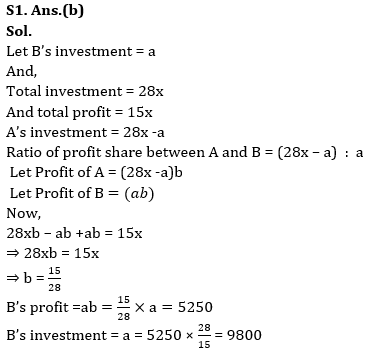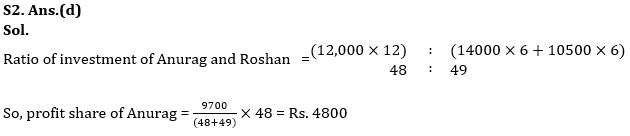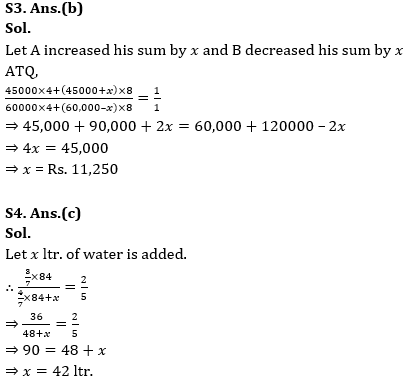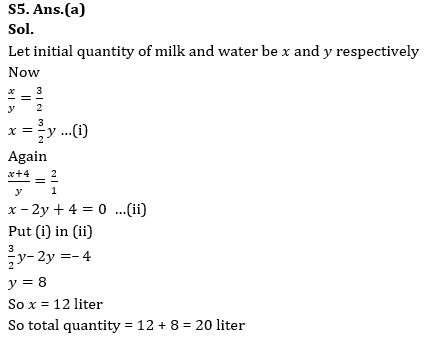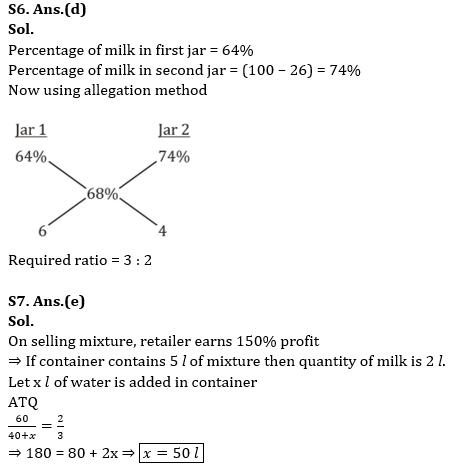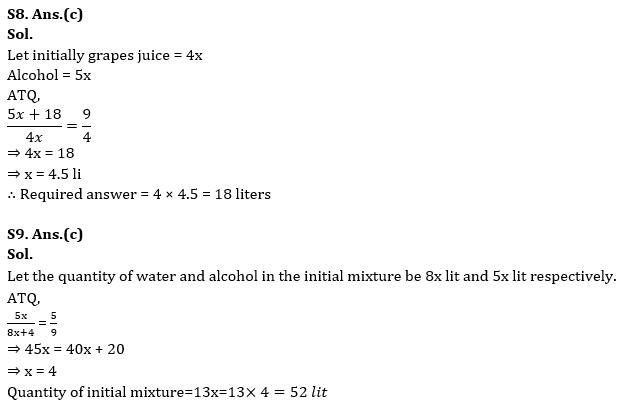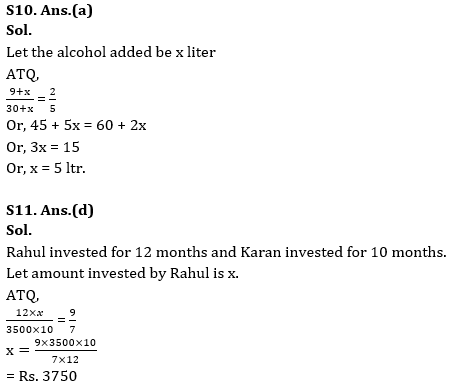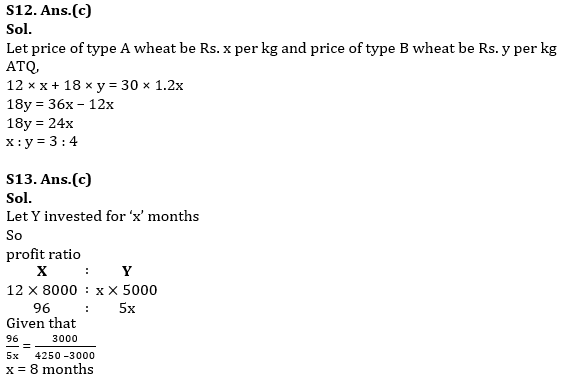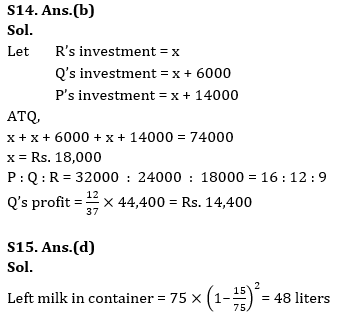.

## FAQs

### What is the selection process of the Bank Clerk?

The selection process of the Bank Clerk is Prelims & Mains.

#### Congratulations!Union Budget 2023-24: Free PDF